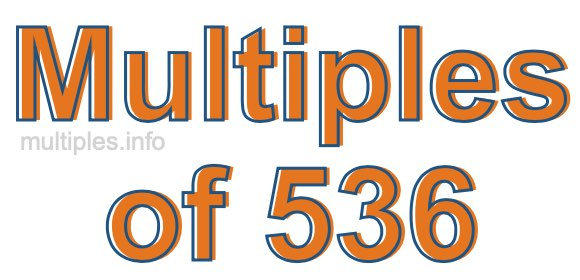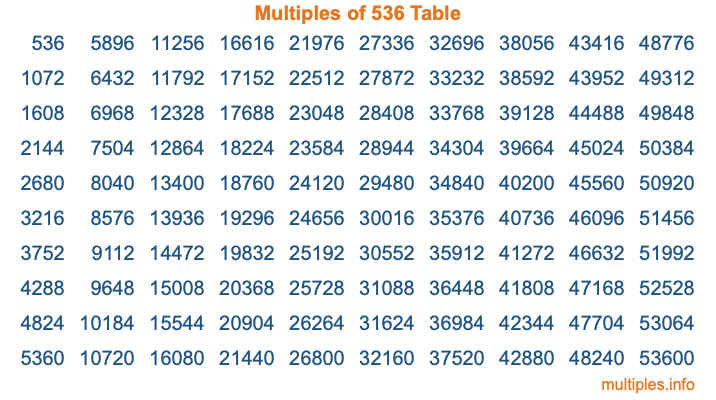Multiples of 536Welcome to the Multiples of 536 page. Here we will first teach you everything you will ever need to know about the multiples of 536, and then give you a study guide summary of everything we taught you to make sure you remember it all. Use this page to look up facts and learn information about the multiples of 536. This page will make you a multiples of five hundred thirty-six expert!

Definition of Multiples of 536
Multiples of 536 are all the numbers that when divided by 536 equal an integer. Each of the multiples of 536 are called a multiple. A multiple of 536 is created by multiplying 536 by an integer.

Therefore, to create a list of multiples of 536, you start with 1 multiplied by 536, then 2 multiplied by 536, then 3 multiplied by 536, and so on for as long as you want. Thus, the list of the first five multiples of 536 is 536, 1072, 1608, 2144, and 2680. To see a larger list of multiples of 536, see the printable image of Multiples of 536 further down on this page. We also have a category where you can choose any nth multiple of 536.

Multiples of 536 Checker
The Multiples of 536 Checker below checks to see if any number of your choice is a multiple of 536. In other words, it checks to see if there is any number (integer) that when multiplied by 536 will equal your number. To do that, we divide your number by 536. If the the quotient is an integer, then your number is a multiple of 536.

Is  a multiple of 536?

Least Common Multiple of 536 and ...
A Least Common Multiple (LCM) is the lowest multiple that two or more numbers have in common. This is also called the smallest common multiple or lowest common multiple and is useful to know when you are adding our subtracting fractions. Enter one or more numbers below (536 is already entered) to find the LCM.

Check out our LCM Calculator if you need more details about the Least Common Multiple or if you need the LCM for different numbers for adding and subtraction fractions.

nth Multiple of 536
As we stated above, 536 is the first multiple of 536, 1072 is the second multiple of 536, 1608 is the third multiple of 536, and so on. Enter a number below to find the nth multiple of 536.

th multiple of 536

Multiples of 536 vs Factors of 536
536 is a multiple of 536 and a factor of 536, but that is where the similarities end. All postive multiples of 536 are 536 or greater than 536. All positive factors of 536 are 536 or less than 536.

Below is the beginning list of multiples of 536 and the factors of 536 so you can compare:

Multiples of 536: 536, 1072, 1608, 2144, 2680, etc.

Factors of 536: 1, 2, 4, 8, 67, 134, 268, 536

As you can see, the multiples of 536 are all the numbers that you can divide by 536 to get a whole number. The factors of 536, on the other hand, are all the whole numbers that you can multiply by another whole number to get 536.

It's also interesting to note that if a number (x) is a factor of 536, then 536 will also be a multiple of that number (x).

Multiples of 536 vs Divisors of 536
The divisors of 536 are all the integers that 536 can be divided by evenly. Below is a list of the divisors of 536.

Divisors of 536: 1, 2, 4, 8, 67, 134, 268, 536

The interesting thing to note here is that if you take any multiple of 536 and divide it by a divisor of 536, you will see that the quotient is an integer.

Multiples of 536 Table
Below is an image of the first 100 multiples of 536 in a table. The table is in chronological order, column by column. The first column has the first ten multiples of 536, the second column has the next ten multiples of 536, and so on.The Multiples of 536 Table is also referred to as the 536 Times Table or Times Table of 536. You are welcome to print out our table for your studies.

Negative Multiples of 536
Although not often discussed or needed in math, it is worth mentioning that you can make a list of negative multiples of 536 by multiplying 536 by -1, then by -2, then by -3, and so on, to get the following list of negative multiples of 536:

-536, -1072, -1608, -2144, -2680, etc.

Multiples of 536 Summary
Below is a summary of important Multiples of 536 facts that we have discussed on this page. To retain the knowledge on this page, we recommend that you read through the summary and explain to yourself or a study partner why they hold true.

There are an infinite number of multiples of 536.

A multiple of 536 divided by 536 will equal a whole number.

536 divided by a factor of 536 equals a divisor of 536.

The nth multiple of 536 is n times 536.

The largest factor of 536 is equal to the first positive multiple of 536.

536 is a multiple of every factor of 536.

536 is a multiple of 536.

A multiple of 536 divided by a divisor of 536 equals an integer.

536 divided by a divisor of 536 equals a factor of 536.

Any integer times 536 will equal a multiple of 536.

Multiples of a Number
Here you can get the multiples of another number, all with the same attention to detail as we did for multiples of 536 on this page.

Multiples of
Multiples of 537
Did you find our page about multiples of five hundred thirty-six educational? Do you want more knowledge? Check out the multiples of the next number on our list!